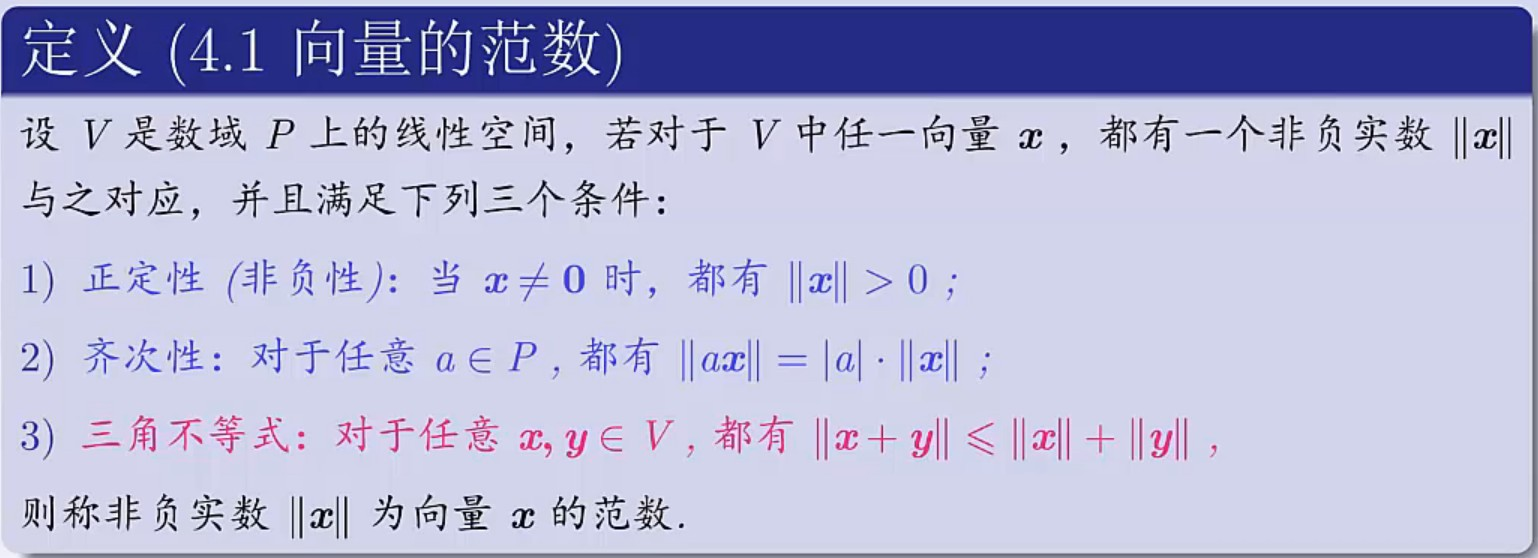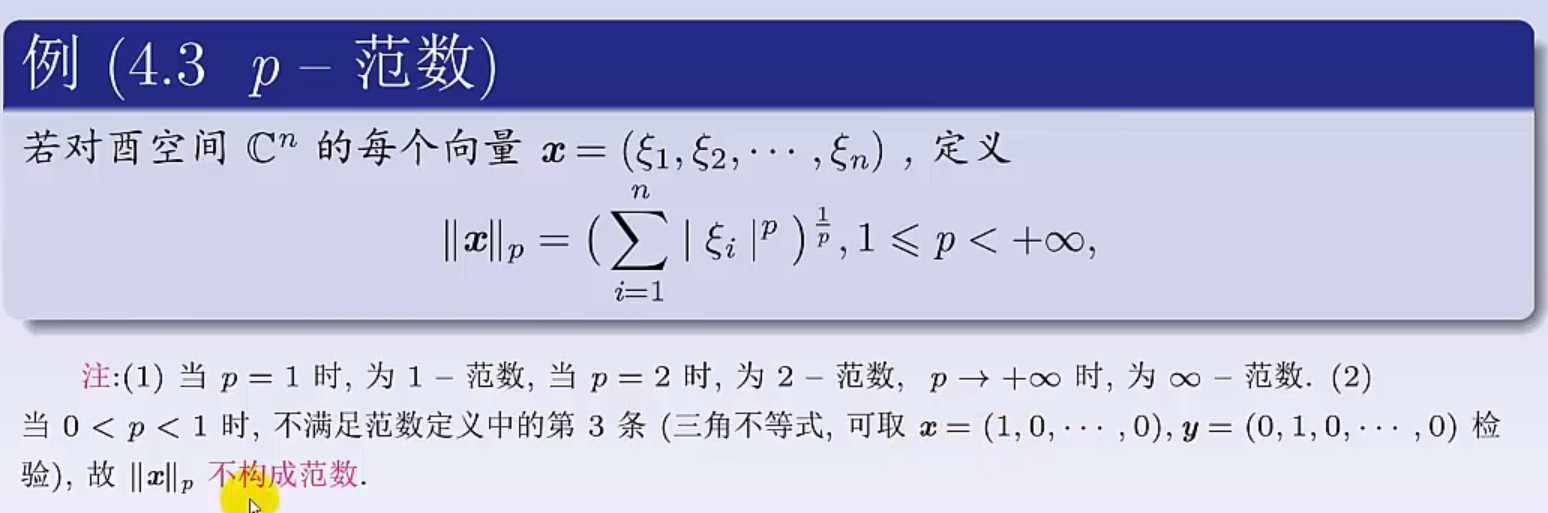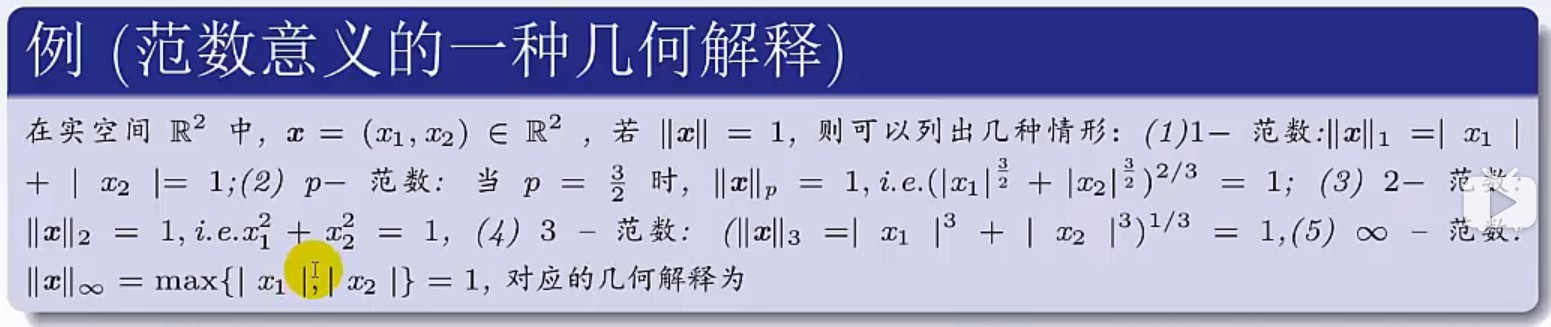Mrli

Contacts:
QQ博客园

# 范数的概念_MATLAB使用

2020/10/27
Word count: 812 | Reading time: 4min

# 范数

## 向量的范数

### 定义## 矩阵的范数▲以上概念来自于B站视频: 向量范数矩阵范数

### Matlab中的norm

demo代码中有关norm的运用如下,

#### 1、如果A为矩阵

返回A的最大奇异值，即max(svd(A))

• n=norm(A,p)

根据p的不同，返回不同的值

p 返回值
1 返回A中最大一列和，即max(sum(abs(A)))
2 返回A的最大奇异值，和n=norm(A)用法一样
inf 返回A中最大一行和，即max(sum(abs(A’)))
‘fro’ A和A‘的积的对角线和的平方根，即sqrt(sum(diag(A’*A)))

#### 2、如果A为向量

• norm(A,p)

返回向量A的p范数。即返回 sum(abs(A).p)(1/p),对任意 1<p<+∞.

• norm(A)

返回向量A的2范数，即等价于norm(A,2)。

• norm(A,inf)

返回max(abs(A))

• norm(A,-inf)

返回min(abs(A))

### Matlab中的sum

• sum(A, 1)： 对一维求和， 如果A为二维数组， 则按列求和
• sum(A, 2): 对二维求和， 如果A为二维数组， 则按行求和

### Matlab中的wgn

white Gaussian noise

Author: Mrli